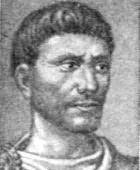# Diophantus quotes3   4

## Diophantus

Diophantus of Alexandria was an Alexandrian Hellenistic mathematician, who was the author of a series of books called Arithmetica, many of which are now lost. His texts deal with solving algebraic equations. While reading Claude Gaspard Bachet de Méziriac's edition of Diophantus' Arithmetica, Pierre de Fermat concluded that a certain equation considered by Diophantus had no solutions, and noted in the margin without elaboration that he had found "a truly marvelous proof of this proposition," now referred to as Fermat's Last Theorem. This led to tremendous advances in number theory, and the study of Diophantine equations and of Diophantine approximations remain important areas of mathematical research. Diophantus coined the term παρισότης to refer to an approximate equality. This term was rendered as adaequalitas in Latin, and became the technique of adequality developed by Pierre de Fermat to find maxima for functions and tangent lines to curves. Diophantus was the first Greek mathematician who recognized fractions as numbers; thus he allowed positive rational numbers for the coefficients and solutions. In modern use, Diophantine equations are usually algebraic equations with integer coefficients, for which integer solutions are sought. Wikipedia

Diophantus

### „If we arrive at an equation containing on each side the same term but with different coefficients, we must take equals from equals until we get one term equal to another term. But, if there are on one or on both sides negative terms, the deficiencies must be added on both bides until all the terms on both sides are positive. Then we must take equals from equals until one term is left on each side.“

As quoted by Thomas Little Heath, Diophantos of Alexandria: https://books.google.com/books?id=ABkPAAAAIAAJ A Study in the History of Greek Algebra (1885)
Arithmetica (c. 250 AD)

### „Perhaps the topic [of this book] will appear fairly difficult to you because it is not yet familiar knowledge and the understanding of beginners is easily confused by mistakes; but with your inspiration and my teaching it will be easy for you to master, because clear intelligence supported by good lessons is a fast route to knowledge.“

Following the dedication to Dionysus as quoted by Paul Drijvers, Secondary Algebra Education (2011)
Arithmetica (c. 250 AD)

### „As a square number is known to be the product of a number multiplied by itself, so every polygonal number, multiplied by one number and added to another, both of which depend upon the number of its angles, produces a square number. I shall prove this, and shall show also how from a given side to find its polygon and conversely. Some auxiliary propositions must first be proved.“

As quoted by James Gow, A Short History of Greek Mathematics https://books.google.com/books?id=9d8DAAAAMAAJ (1884)
Arithmetica (c. 250 AD)

### Similar authors

##### Pythagoras121
ancient Greek mathematician and philosopher
##### Thales8
ancient Greek philosopher and mathematician
##### Archimedes19
Greek mathematician, physicist, engineer, inventor, and ast…
Greek poet
##### Giorgos Seferis2
Greek poet and diplomat
##### Plutarch249
ancient Greek historian and philosopher
##### Antisthenes24
Greek philosopher
##### Anacreon12
Greek lyric poet, notable for his drinking songs and hymns
##### Xenophon18
ancient Greek historian and philosopher
##### Homér217
Ancient Greek epic poet, author of the Iliad and the Odyssey